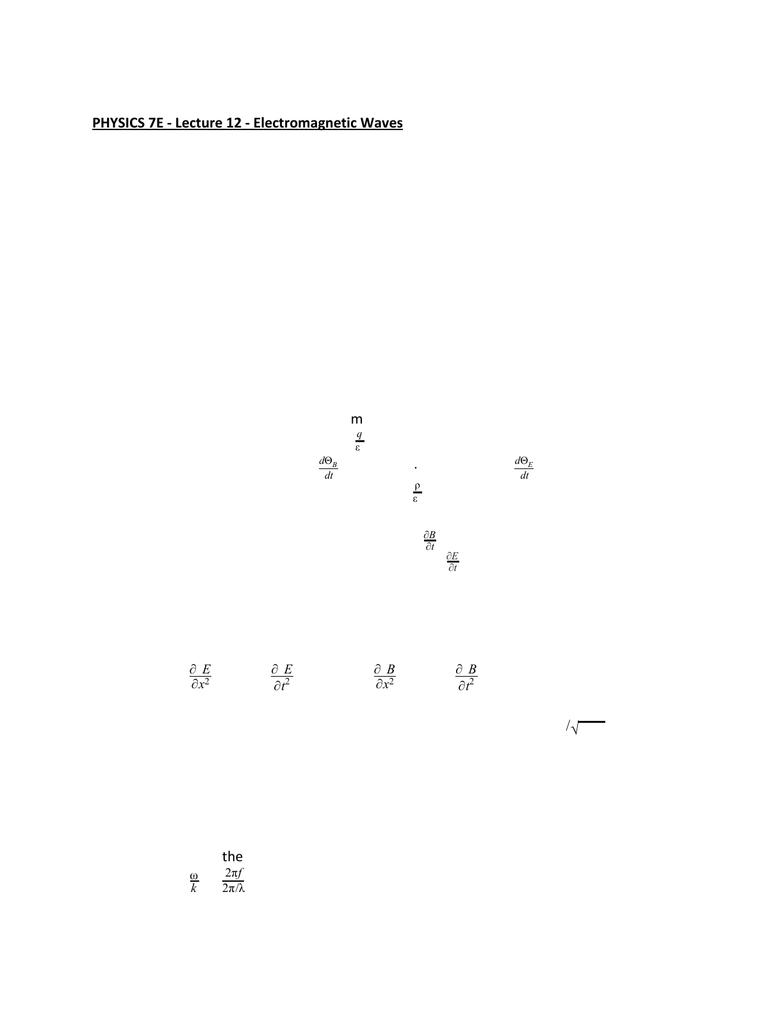Class Notes (1,100,000)
US (460,000)
UC-Irvine (10,000)
PHYSICS (500)
Lecture 12

# PHYSICS 7E Lecture Notes - Lecture 12: Partial Differential Equation, Electric Flux, Electric FieldPremium

Department
Physics
Course Code
PHYSICS 7E
Professor
GUERRA, A
Lecture
12

This preview shows half of the first page. to view the full 3 pages of the document.11/05/19
PHYSICS 7E - Lecture 12 - Electromagnetic Waves
Electromagnetic Waves
Electromagnetic (em) waves permeate our environment
EM waves can propagate through a vacuum
Much of the behavior of mechanical wave models is similar for em waves
Maxwell’s equations form the basis of all electromagnetic phenomena
Maxwell’s Equation
In his unified theory of electromagnetism, Maxwell showed that electromagnetic waves
are a natural consequence of the fundamental laws expressed in these four equations:
Gauss’ Law (electric flux)
Gauss’ Law for magnetism
Ampere’s Law, General form
AE ·d=q
ε0
AB ·d= 0
sE ·d= dt
dΘBs l εB·d= μ0+ μ0 0 dt
dΘE
·E=ρ
ε0
·B= 0
×E= t
B
ε J×B= μ0+ μ0 0 t
E
Derivation of Speed of EM Wave
From Maxwell’s equations applied to empty space, the following partial derivatives can
be found:
ε
x2
E
2= μoot2
E
2
and ε
x2
B
2= μoot2
B
2
The simplest solution to the partial differential equations is a sinusoidal wave:
cos(kx t)E=Emax − ω /v=c= 1 μ ε
oo
cos(kx t)B=Bmax − ω
These are in the form of a general wave equation, with
Substituting the values for and gives m/sμoεo.99792 10c= 2 × 8
E, B, and C
The speed of the electromagnetic wave is
f
k
ω=2πf
2π/λ = λ = c
Taking partial derivatives also gives